# Lecture 18 – Data 100, Fall 2020¶

by Suraj Rampure

adapted from Josh Hug, Joseph Gonzalez

In :
import numpy as np
import pandas as pd
import matplotlib.pyplot as plt
import seaborn as sns
import plotly.express as px
import plotly.graph_objs as go
from scipy.optimize import minimize
import sklearn.linear_model as lm

# plt.rcParams['figure.figsize'] = (4, 4)
plt.rcParams['figure.dpi'] = 150
plt.rcParams['lines.linewidth'] = 3
sns.set()


## Motivating Logistic Regression¶

In this lecture, we will look at data from the 2017-18 NBA season.

In :
df = pd.read_csv('nba.csv')

In :
df.head()

Out:
SEASON_ID TEAM_ID TEAM_ABBREVIATION TEAM_NAME GAME_ID GAME_DATE MATCHUP WL MIN FGM ... DREB REB AST STL BLK TOV PF PTS PLUS_MINUS VIDEO_AVAILABLE
0 22017 1610612744 GSW Golden State Warriors 21700002 2017-10-17 GSW vs. HOU L 240 43 ... 35 41 34 5 9 17 25 121 -1 1
1 22017 1610612745 HOU Houston Rockets 21700002 2017-10-17 HOU @ GSW W 240 47 ... 33 43 28 9 5 13 16 122 1 1
2 22017 1610612738 BOS Boston Celtics 21700001 2017-10-17 BOS @ CLE L 240 36 ... 37 46 24 11 4 12 24 99 -3 1
3 22017 1610612739 CLE Cleveland Cavaliers 21700001 2017-10-17 CLE vs. BOS W 240 38 ... 41 50 19 3 4 17 25 102 3 1
4 22017 1610612750 MIN Minnesota Timberwolves 21700011 2017-10-18 MIN @ SAS L 240 37 ... 31 42 23 7 4 13 16 99 -8 1

5 rows × 29 columns

We are eventually going to want to perform binary classification, which is where we predict a 1 or 0. A reasonable thing to want to do given this data is to predict whether or not a team wins. Right now, the WL column consists of "W" and "L".

In :
df['WL']

Out:
0       L
1       W
2       L
3       W
4       L
..
2455    W
2456    W
2457    L
2458    W
2459    L
Name: WL, Length: 2460, dtype: object

Let's fix that, so that wins are encoded as 1 and losses are encoded as 0.

In :
df["WON"] = df["WL"]
df["WON"] = df["WON"].replace("W", 1)
df["WON"] = df["WON"].replace("L", 0)

Out:
SEASON_ID TEAM_ID TEAM_ABBREVIATION TEAM_NAME GAME_ID GAME_DATE MATCHUP WL MIN FGM ... REB AST STL BLK TOV PF PTS PLUS_MINUS VIDEO_AVAILABLE WON
0 22017 1610612744 GSW Golden State Warriors 21700002 2017-10-17 GSW vs. HOU L 240 43 ... 41 34 5 9 17 25 121 -1 1 0
1 22017 1610612745 HOU Houston Rockets 21700002 2017-10-17 HOU @ GSW W 240 47 ... 43 28 9 5 13 16 122 1 1 1
2 22017 1610612738 BOS Boston Celtics 21700001 2017-10-17 BOS @ CLE L 240 36 ... 46 24 11 4 12 24 99 -3 1 0
3 22017 1610612739 CLE Cleveland Cavaliers 21700001 2017-10-17 CLE vs. BOS W 240 38 ... 50 19 3 4 17 25 102 3 1 1
4 22017 1610612750 MIN Minnesota Timberwolves 21700011 2017-10-18 MIN @ SAS L 240 37 ... 42 23 7 4 13 16 99 -8 1 0

5 rows × 30 columns

There is a row for each team and each game in this dataset. It contains the FG_PCT (field goal percentage) for each team per game.

In :
df['FG_PCT']

Out:
0       0.538
1       0.485
2       0.409
3       0.458
4       0.435
...
2455    0.472
2456    0.553
2457    0.484
2458    0.591
2459    0.402
Name: FG_PCT, Length: 2460, dtype: float64

Let's try and get the field goal percentage difference between two teams in a single game. We will then try and use this value to predict whether or not a team wins, given their field goal percentage difference.

This data cleaning and EDA is not the point of this lecture, but you may want to come back to this and try and understand it.

In :
one_team = df.groupby("GAME_ID").first()
opponent = df.groupby("GAME_ID").last()
games = one_team.merge(opponent, left_index = True, right_index = True, suffixes = ["", "_OPP"])
games["FG_PCT_DIFF"] = games["FG_PCT"] - games["FG_PCT_OPP"]
games['WON'] = games['WL'].replace('L', 0).replace('W', 1)
games = games[['TEAM_NAME', 'MATCHUP', 'WON', 'FG_PCT_DIFF']]

In :
games.head()

Out:
TEAM_NAME MATCHUP WON FG_PCT_DIFF
GAME_ID
21700001 Boston Celtics BOS @ CLE 0 -0.049
21700002 Golden State Warriors GSW vs. HOU 0 0.053
21700003 Charlotte Hornets CHA @ DET 0 -0.030
21700004 Indiana Pacers IND vs. BKN 1 0.041
21700005 Orlando Magic ORL vs. MIA 1 0.042

Let's start by looking at a sns.jointplot of FG_PCT_DIFF and WON.

In :
sns.jointplot(data = games, x = "FG_PCT_DIFF", y = "WON");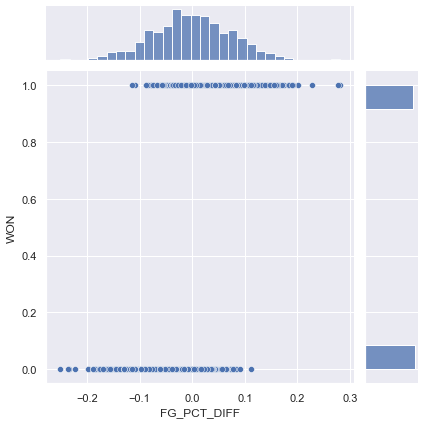A reasonable thing to do here might be to model the probability of winning, given FG_PCT_DIFF.

We already know how to use ordinary least squares, right? Why not use it here?

We'll also jitter the data, to get a better picture of what it looks like. But the line of best fit that's being drawn is on top of the original, non-jittered data.

In :
sns.jointplot(data = games, x = "FG_PCT_DIFF", y = "WON",
y_jitter = 0.1,
kind="reg",
ci=False,
joint_kws={'line_kws':{'color':'green'}});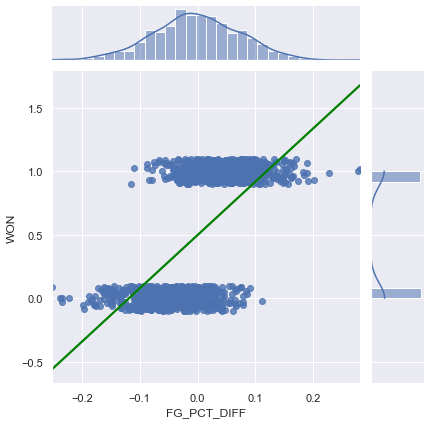The green line drawn is a valid model. It is the line that minimizes MSE for this set of $x$ (FG_PCT_DIFF) and $y$ (WON) data.

But there are some issues:

• The outputs are bigger than 0 and less than 1. How do we interpret that?
• This is very susceptible to outliers. See:
In :
games2 = games.copy()

In :
games2.iloc = ['hello', 'hello', 1, 120]

In :
sns.jointplot(data = games2, x = "FG_PCT_DIFF", y = "WON",
y_jitter = 0.1,
kind="reg",
ci=False,
joint_kws={'line_kws':{'color':'green'}});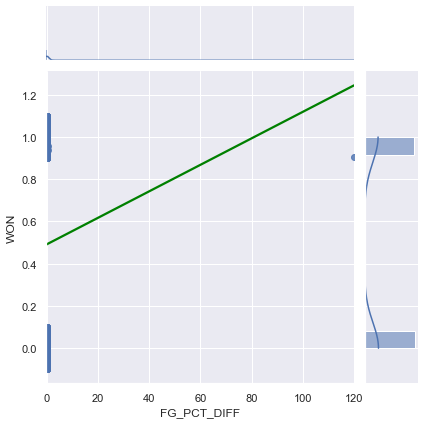We need a better model. Let's try and replicate the graph of averages from Lecture 12, on Simple Linear Regression. Recall, we

• binned the $x$ axis.
• took the average $y$ value for each bin on the $x$ axis.

We will do the same thing here, albeit with slightly different code. Here, we will formally partition the $x$-axis into 20 bins.

In :
bins = pd.cut(games["FG_PCT_DIFF"], 20)

In :
bins

Out:
GAME_ID
21700001    (-0.0648, -0.0382]
21700002      (0.0416, 0.0682]
21700003    (-0.0382, -0.0116]
21700004       (0.015, 0.0416]
21700005      (0.0416, 0.0682]
...
21701226        (0.175, 0.201]
21701227      (0.0682, 0.0948]
21701228       (0.015, 0.0416]
21701229    (-0.0914, -0.0648]
21701230     (-0.118, -0.0914]
Name: FG_PCT_DIFF, Length: 1230, dtype: category
Categories (20, interval[float64]): [(-0.252, -0.224] < (-0.224, -0.198] < (-0.198, -0.171] < (-0.171, -0.145] ... (0.175, 0.201] < (0.201, 0.228] < (0.228, 0.254] < (0.254, 0.281]]
In :
games["bin"] = [(b.left + b.right) / 2 for b in bins]
games["bin"]

Out:
GAME_ID
21700001   -0.0515
21700002    0.0549
21700003   -0.0249
21700004    0.0283
21700005    0.0549
...
21701226    0.1880
21701227    0.0815
21701228    0.0283
21701229   -0.0781
21701230   -0.1047
Name: bin, Length: 1230, dtype: float64
In :
games

Out:
TEAM_NAME MATCHUP WON FG_PCT_DIFF bin
GAME_ID
21700001 Boston Celtics BOS @ CLE 0 -0.049 -0.0515
21700002 Golden State Warriors GSW vs. HOU 0 0.053 0.0549
21700003 Charlotte Hornets CHA @ DET 0 -0.030 -0.0249
21700004 Indiana Pacers IND vs. BKN 1 0.041 0.0283
21700005 Orlando Magic ORL vs. MIA 1 0.042 0.0549
... ... ... ... ... ...
21701226 New Orleans Pelicans NOP vs. SAS 1 0.189 0.1880
21701227 Oklahoma City Thunder OKC vs. MEM 1 0.069 0.0815
21701228 LA Clippers LAC vs. LAL 0 0.017 0.0283
21701229 Utah Jazz UTA @ POR 0 -0.090 -0.0781
21701230 Houston Rockets HOU @ SAC 0 -0.097 -0.1047

1230 rows × 5 columns

We now know which "bin" each game belongs to. We can plot the average WON for each bin.

In :
win_rates_by_bin = games.groupby("bin")["WON"].mean()
win_rates_by_bin

Out:
bin
-0.2380    0.000000
-0.2110    0.000000
-0.1845    0.000000
-0.1580    0.000000
-0.1315    0.000000
-0.1047    0.033898
-0.0781    0.083333
-0.0515    0.148438
-0.0249    0.363636
0.0017    0.505747
0.0283    0.705128
0.0549    0.792793
0.0815    0.907407
0.1079    0.984615
0.1345    1.000000
0.1615    1.000000
0.1880    1.000000
0.2410    1.000000
0.2675    1.000000
Name: WON, dtype: float64
In :
plt.plot(win_rates_by_bin, 'r')

Out:
[<matplotlib.lines.Line2D at 0x7fec8166d1d0>]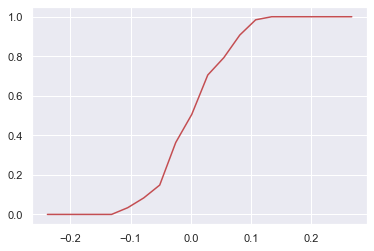In :
sns.jointplot(data = games, x = "FG_PCT_DIFF", y = "WON",
y_jitter = 0.1,
kind="reg",
ci=False,
joint_kws={'line_kws':{'color':'green'}});
plt.plot(win_rates_by_bin, 'r', linewidth = 5);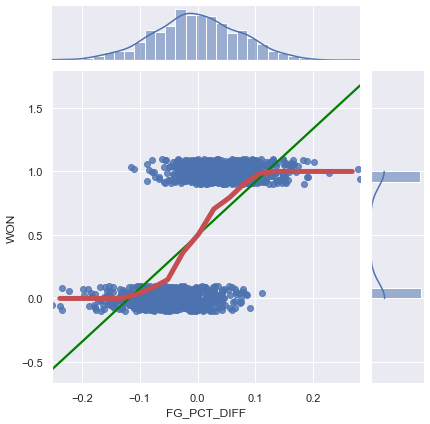It seems like our red graph of averages does a much better job at matching the data than our simple linear regression line.

What is this graph of averages plotting? Since the $y$ axis is only 0s and 1s, and we took the mean of the $y$-values in each bin for a given $x$, the graph of average is plotting the proportion of times a team won, given their FG_PCT_DIFF. Remember, WON = 1 each time a team won.

Logistic regression aims to model the probability of an observation belonging to class 1, given some set of features.

In :
def sigma(t):
return 1 / (1 + np.exp(-t))

In :
plt.plot(win_rates_by_bin, 'r', linewidth = 5);
x = win_rates_by_bin.index
plt.plot(x, sigma(x * 30), 'black', linewidth = 5);
plt.xlabel('FG_PCT_DIFF')
plt.ylabel('WON');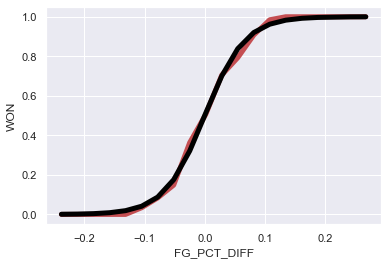What is this mystery sigma function, and why does sigma(x * 30) match our graph of averages so well? Well... we're getting there.

For now, consider these questions:

What are:

1. $P(Y = 1 | X = 0.0283)$?
2. $P(Y = 0 | X = 0.0283)$?
3. $\frac{P(Y = 1 | X = 0.0283)}{P(Y = 0 | X = 0.0283)}$? In other words, how many wins are there for each loss?
In :
win_rates_by_bin

Out:
bin
-0.2380    0.000000
-0.2110    0.000000
-0.1845    0.000000
-0.1580    0.000000
-0.1315    0.000000
-0.1047    0.033898
-0.0781    0.083333
-0.0515    0.148438
-0.0249    0.363636
0.0017    0.505747
0.0283    0.705128
0.0549    0.792793
0.0815    0.907407
0.1079    0.984615
0.1345    1.000000
0.1615    1.000000
0.1880    1.000000
0.2410    1.000000
0.2675    1.000000
Name: WON, dtype: float64

The odds of an event are defined as the probability that it happens divided by the probability that it doesn't happen.

If some event happens with probability $p$, then $\text{odds}(p) = \frac{p}{1-p}$.

In :
odds_by_bin = win_rates_by_bin / (1 - win_rates_by_bin)
odds_by_bin

Out:
bin
-0.2380     0.000000
-0.2110     0.000000
-0.1845     0.000000
-0.1580     0.000000
-0.1315     0.000000
-0.1047     0.035088
-0.0781     0.090909
-0.0515     0.174312
-0.0249     0.571429
0.0017     1.023256
0.0283     2.391304
0.0549     3.826087
0.0815     9.800000
0.1079    64.000000
0.1345          inf
0.1615          inf
0.1880          inf
0.2410          inf
0.2675          inf
Name: WON, dtype: float64

If we plot the odds of these probabilities, they look exponential:

In :
plt.plot(odds_by_bin);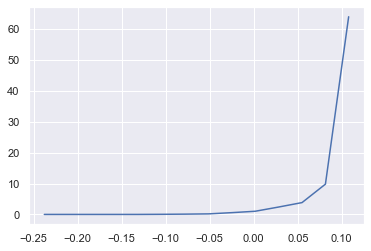But if we take the log of these odds:

In :
plt.plot(np.log(odds_by_bin));

/opt/anaconda3/lib/python3.7/site-packages/pandas/core/series.py:853: RuntimeWarning:

divide by zero encountered in log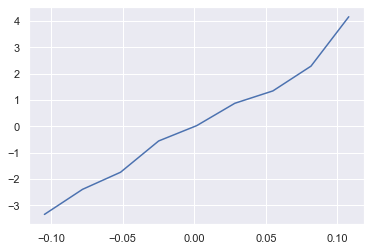We noticed that the log-odds grows linearly with $x$.

In the lecture slides, we formalize what this means, and how this allows us to arrive at the sigma function above.

## The Logistic Function¶

In the slides, we show that our model is

$$P(Y = 1 | x) = \sigma(x^T \theta)$$

where $$\sigma(t) = \frac{1}{1 + e^{-t}}$$

Let's explore the shape of the logistic function, $\sigma$.

First, the vanilla curve $\sigma(x)$:

In :
x = np.linspace(-5,5,50)
plt.plot(x, sigma(x));
plt.xlabel('x')
plt.ylabel(r'$\frac{1}{1 + e^{-x}}$');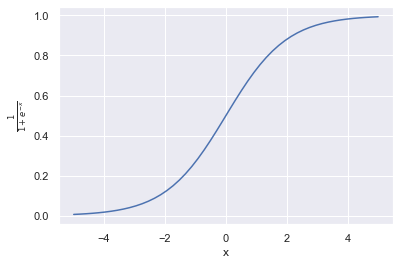Now, we look at $\sigma(\theta_1 x)$, for several values of $\theta_1$:

In :
def flatten(li):
return [item for sub in li for item in sub]

bs = [-2, -1, -0.5, 2, 1, 0.5]
xs = np.linspace(-10, 10, 100)

fig, axes = plt.subplots(2, 3, sharex=True, sharey=True, figsize=(10, 6))
for ax, b in zip(flatten(axes), bs):
ys = sigma(xs * b)
ax.plot(xs, ys)
ax.set_title(r'$\theta_1 =$' + str(b))

# add a big axes, hide frame
# hide tick and tick label of the big axes
plt.tick_params(labelcolor='none', top=False, bottom=False,
left=False, right=False)
plt.grid(False)
plt.xlabel('$x$')
plt.ylabel(r'$\frac{1}{1+\exp(-\theta_1 \cdot x)}$')
plt.tight_layout()
plt.savefig('sigmoids.png')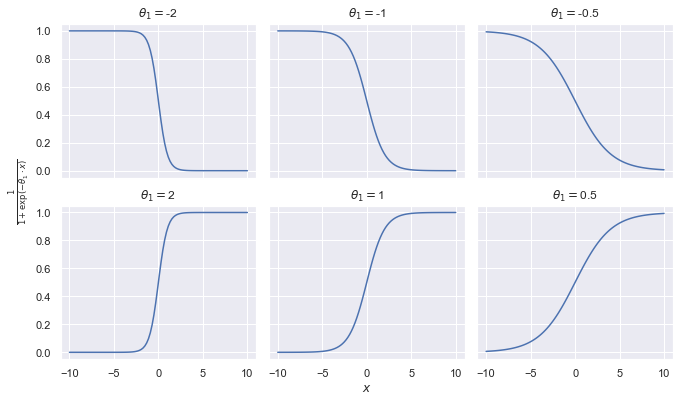Let's explore the shape of $\sigma(\theta_0 + \theta_1x)$, for different values of $\theta_0, \theta_1$. There's quite a bit going on here, so let's use plotly.

In :
fig = go.Figure()
for theta1 in [-1,1, 5]:
for theta0 in [-2, 0, 2]:
fig.add_trace(go.Scatter(name=f"{theta0} + {theta1} x", x=xs, y=sigma(theta0 + theta1*xs)))
fig